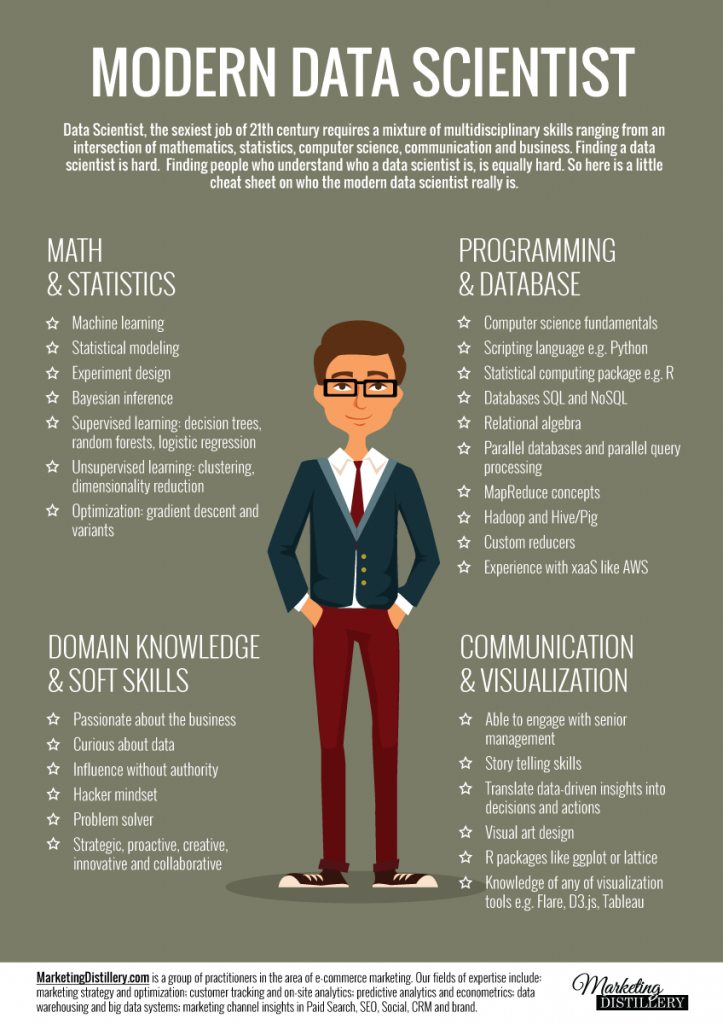(source)

What is data visualization?

• The visual representation of information
• Goals of data visualization
• Effective, clear communication of information
• Stimulate viewer engagement
• Exploratory data analysis

• With many numbers and large datasets, need an efficient way to understand a vast amount of data
• The human visual system is the highest-bandwidth channel to the human brain

Example: Given the income, college degree percentage of each state, try answering the following questions with either a table and a graphic representation. Which method is better in answering the questions?
- Which state has highest income?
- Relationship between income and education?
- Outliers?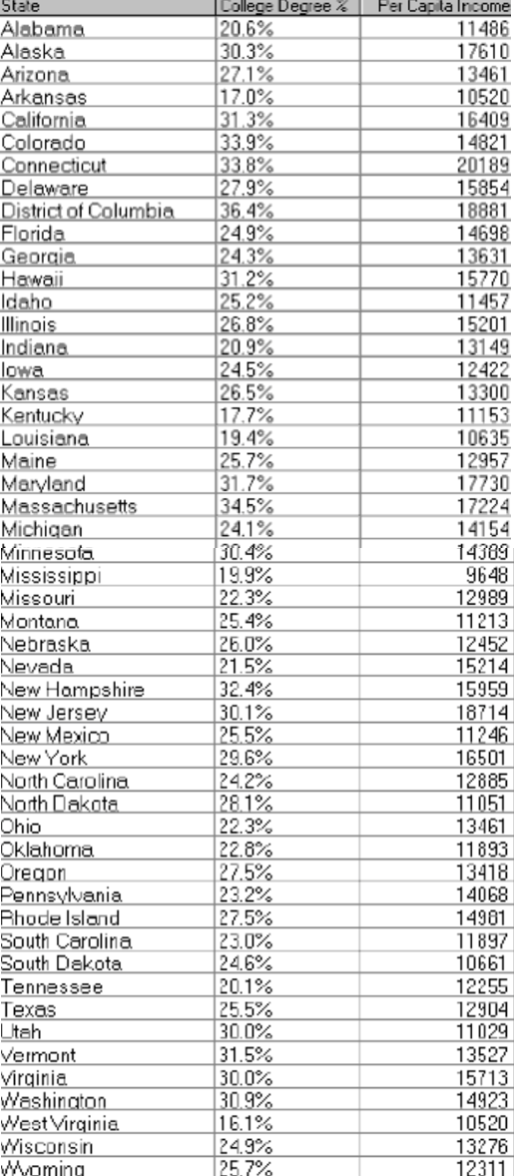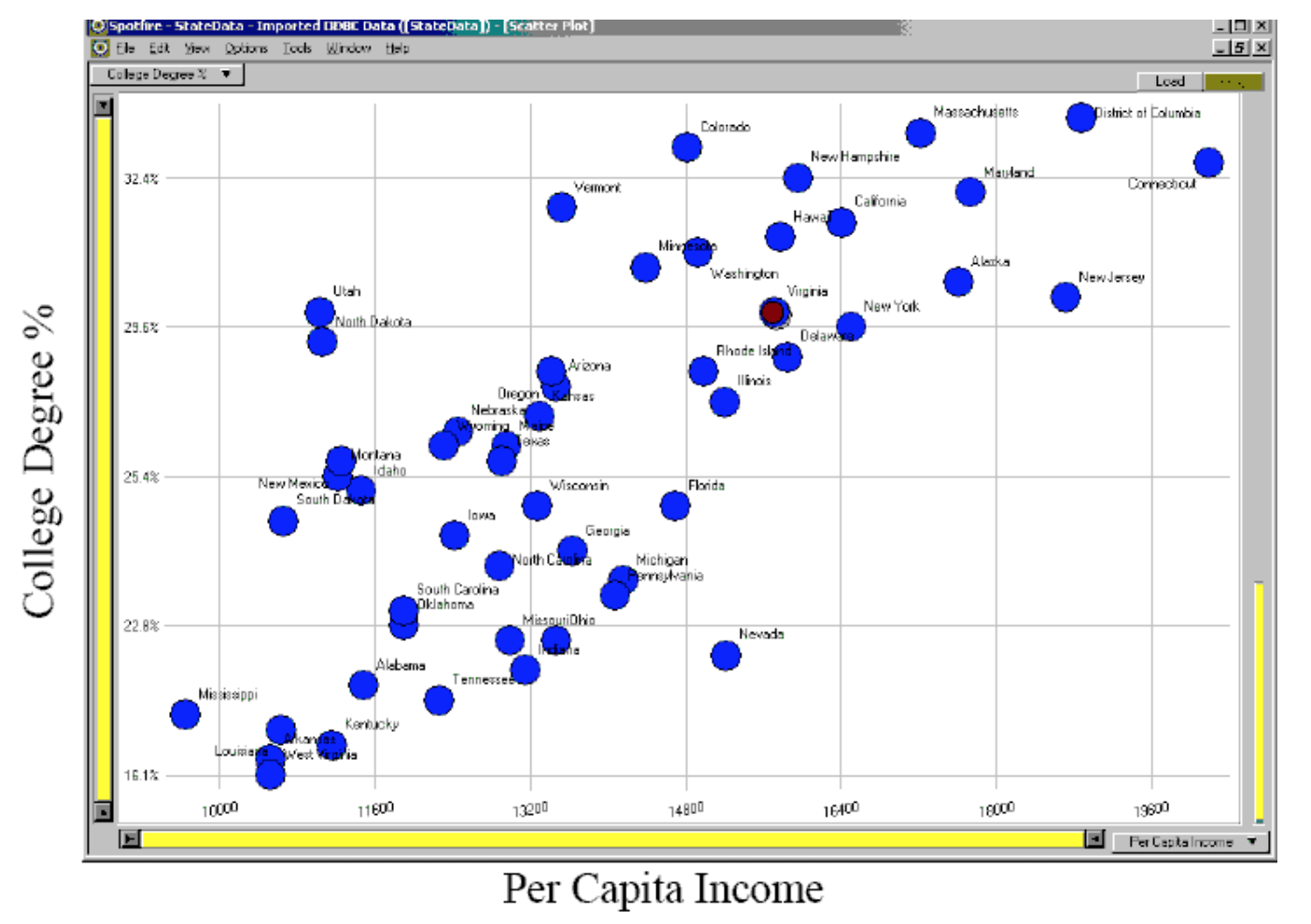(Example by Marti Hearst)

• Graphs reveal data that statistics may not

Example: Anscombe's quartet

IIIIIIIV
xyxyxyxy
10.08.0410.09.1410.07.468.06.58
8.06.958.08.148.06.778.05.76
13.07.5813.08.7413.012.748.07.71
9.08.819.08.779.07.118.08.84
11.08.3311.09.2611.07.818.08.47
14.09.9614.08.1014.08.848.07.04
6.07.246.06.136.06.088.05.25
4.04.264.03.104.05.3919.012.50
12.010.8412.09.1312.08.158.05.56
7.04.827.07.267.06.428.07.91
5.05.685.04.745.05.738.06.89
Simple summary statistics are all identical for four datasets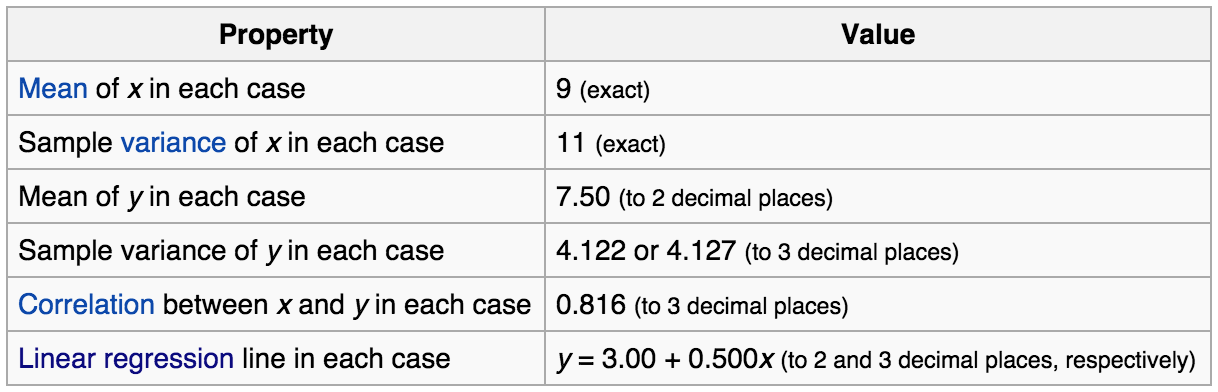However, the four datasets vary considerably when graphed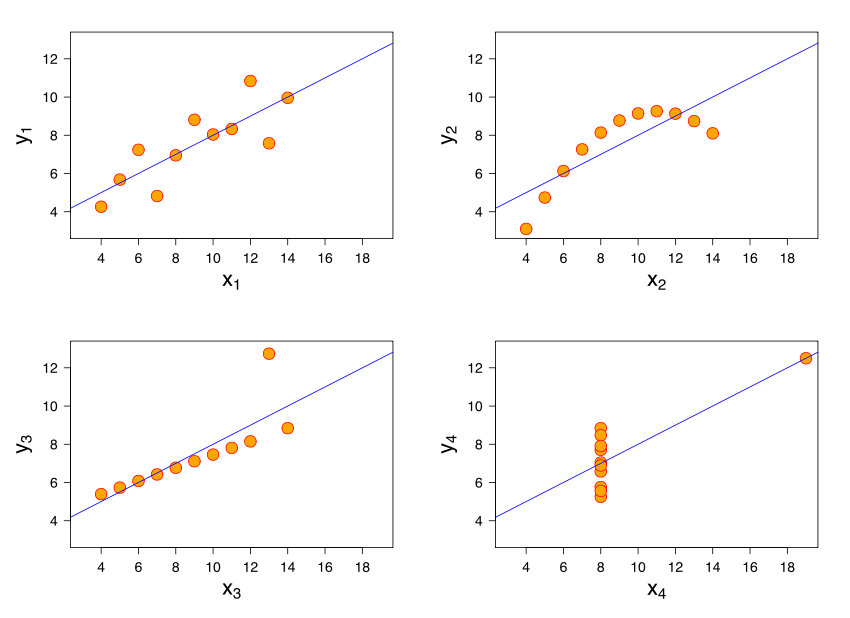Data visualization process

1. Classify datatypes
• Nominal (ex: fruits - apples, oranges, ...)
• Operations: ==, !=
• Ordinal (ex: quality of meat - grade A, AA, AAA, ...)
• Operations: ==, !=, <=, >=
• Quantitative
• Interval (ex: dates - May 1st, 2015, location - LAT 38.9 LON 127)
• Only differences may compared
• Operations: ==, !=, <=, >=, -
• Ratio (ex: length - 160cm)
• Origin is meaningful
• Operations: ==, !=, <=, >=, -, /
2. Map datasets to visual attributes that represent data types most effectively (also known as data encoding)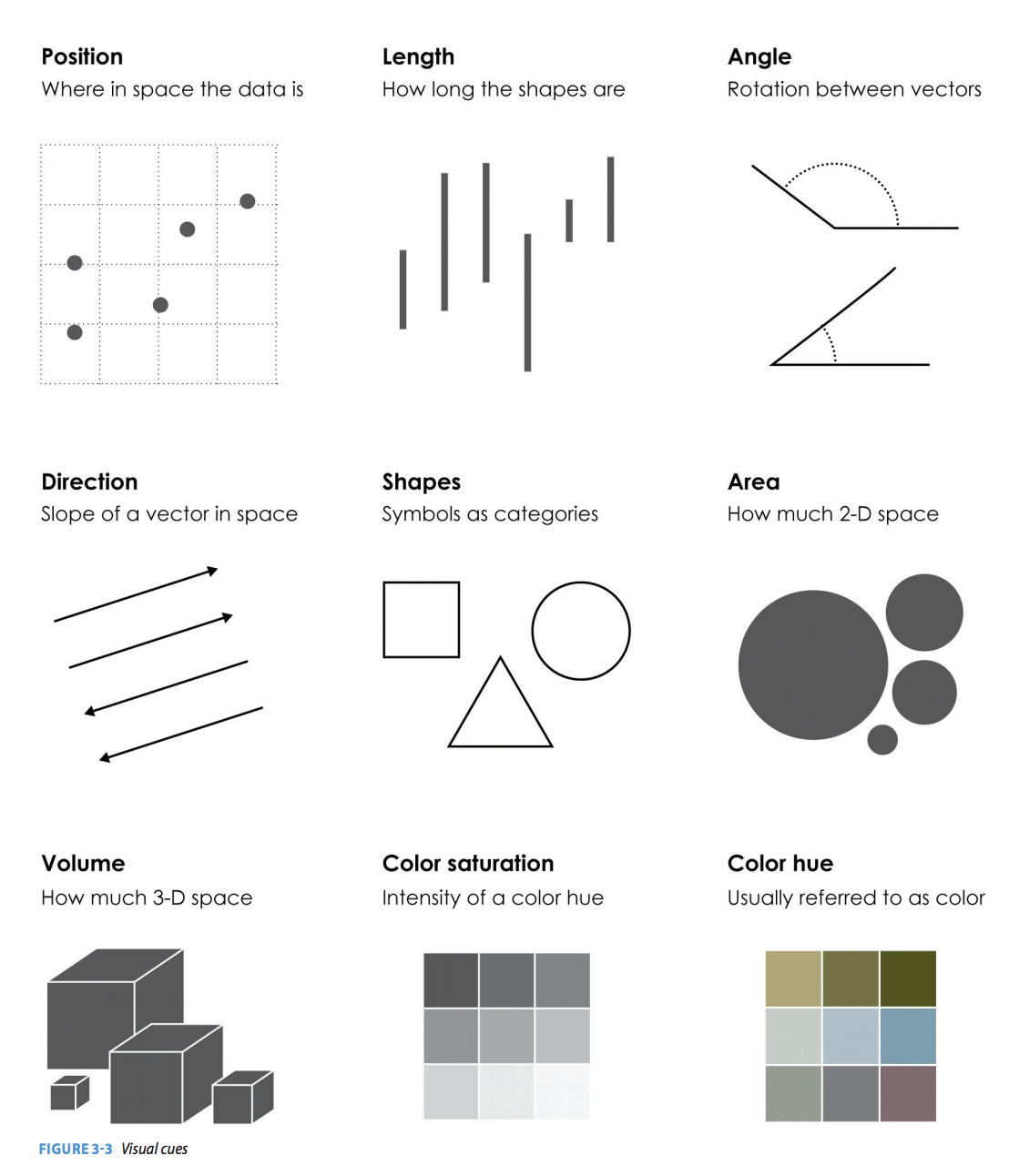3. Encode data to visual variables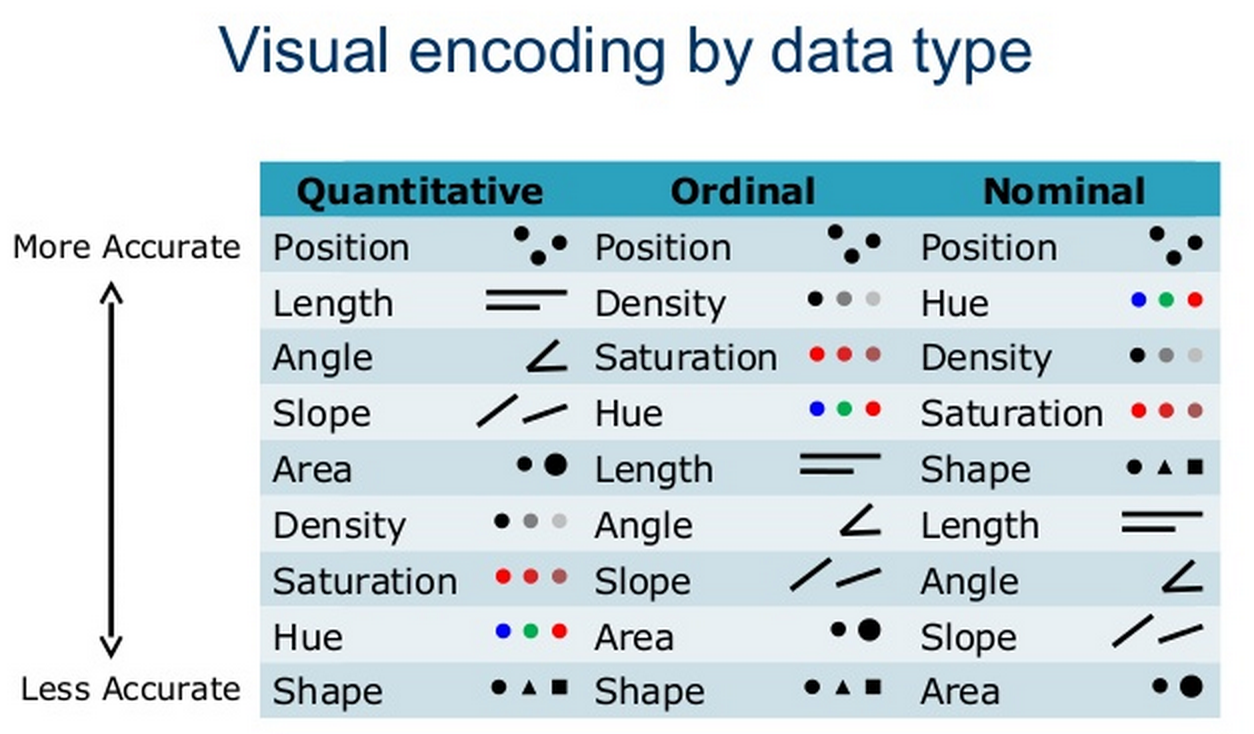4. Comparisons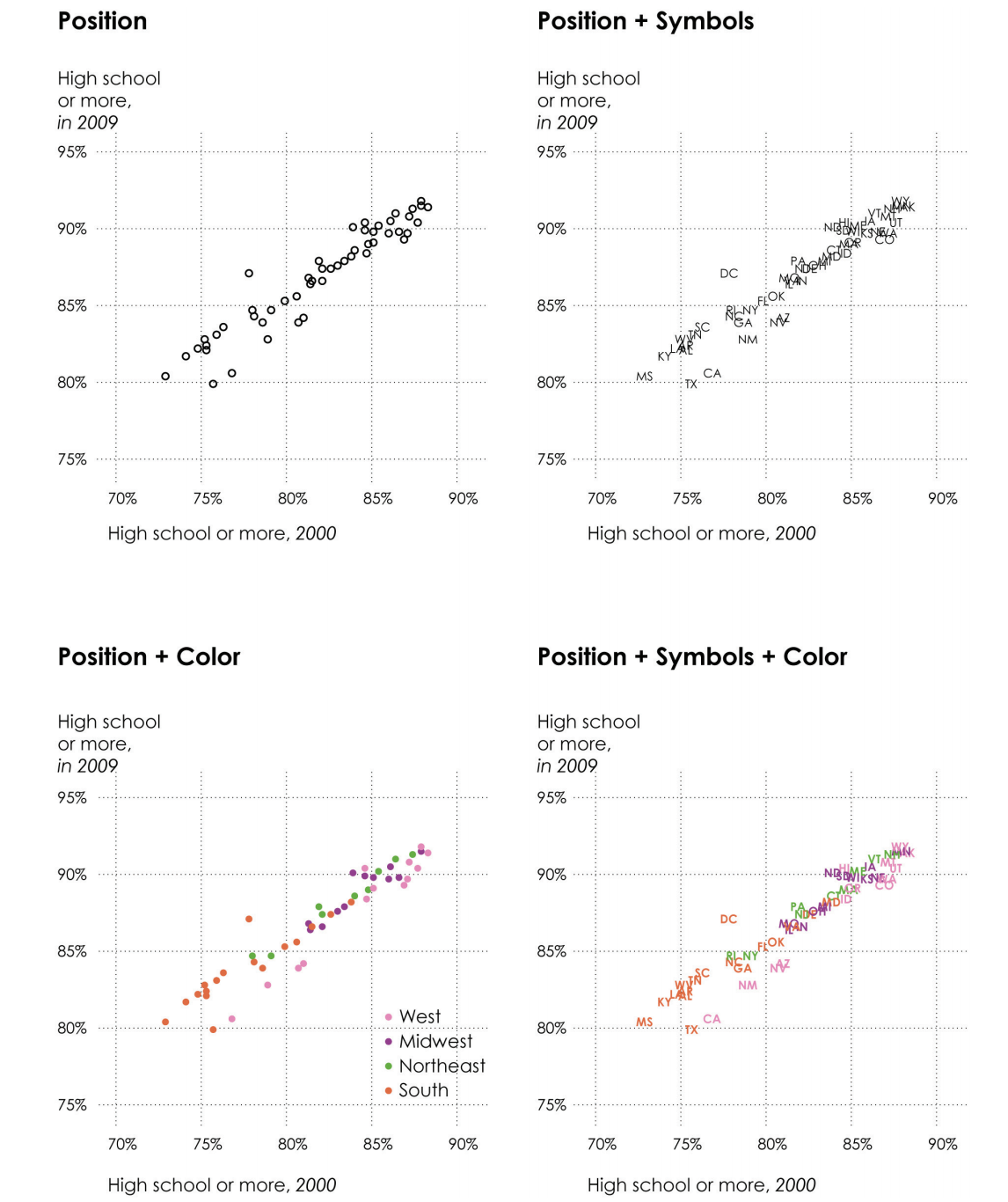(Source: Nathan Yau, Data points)

Data visualization types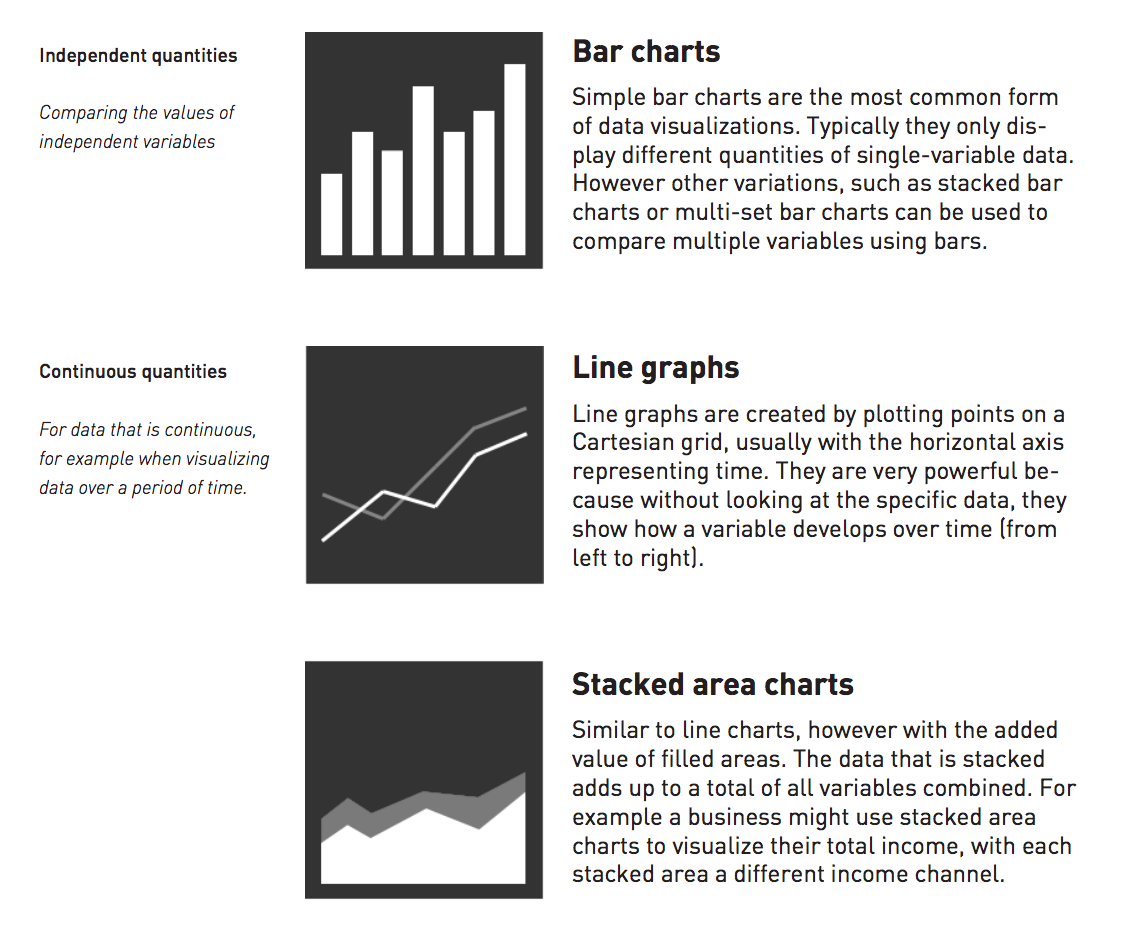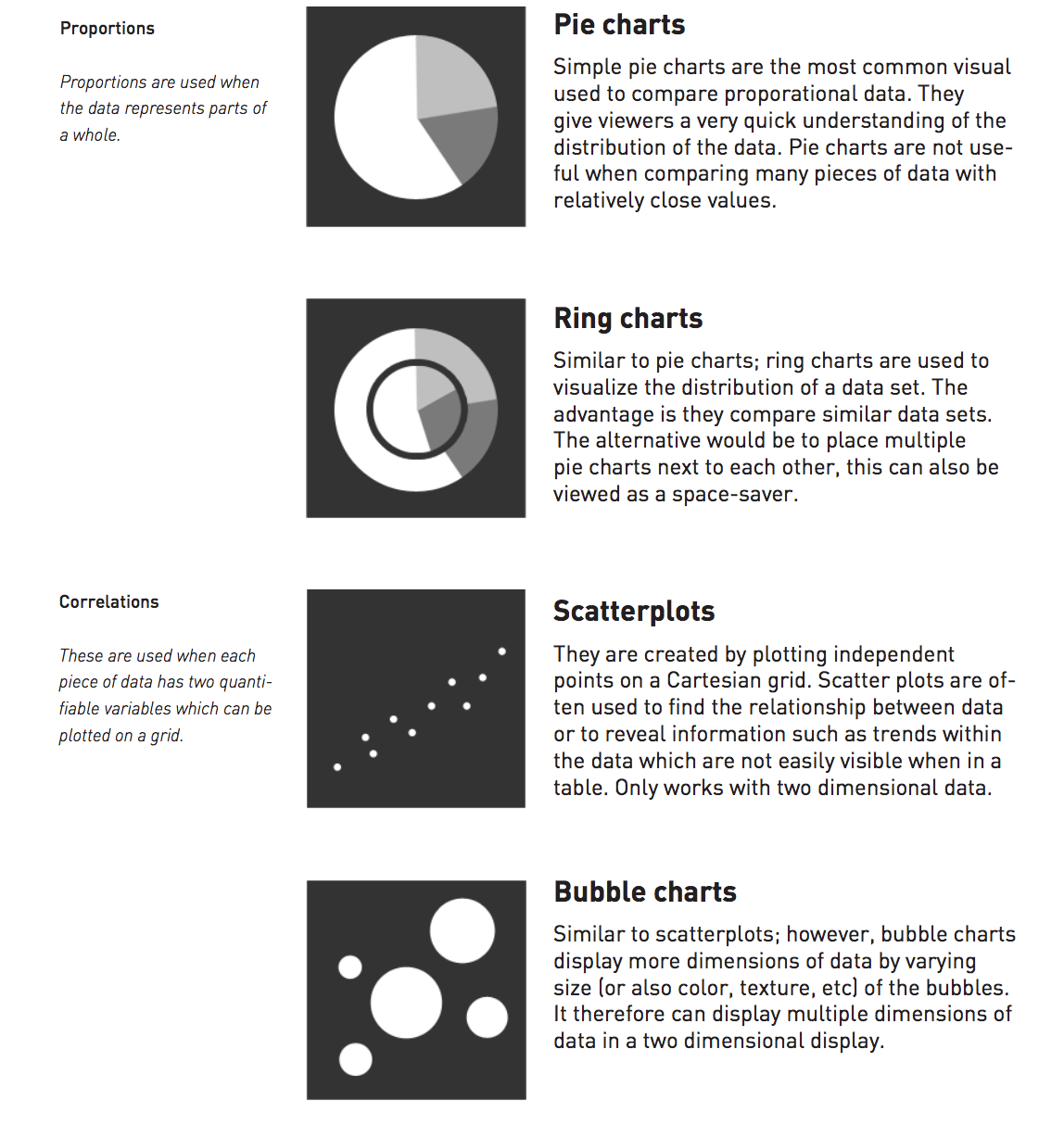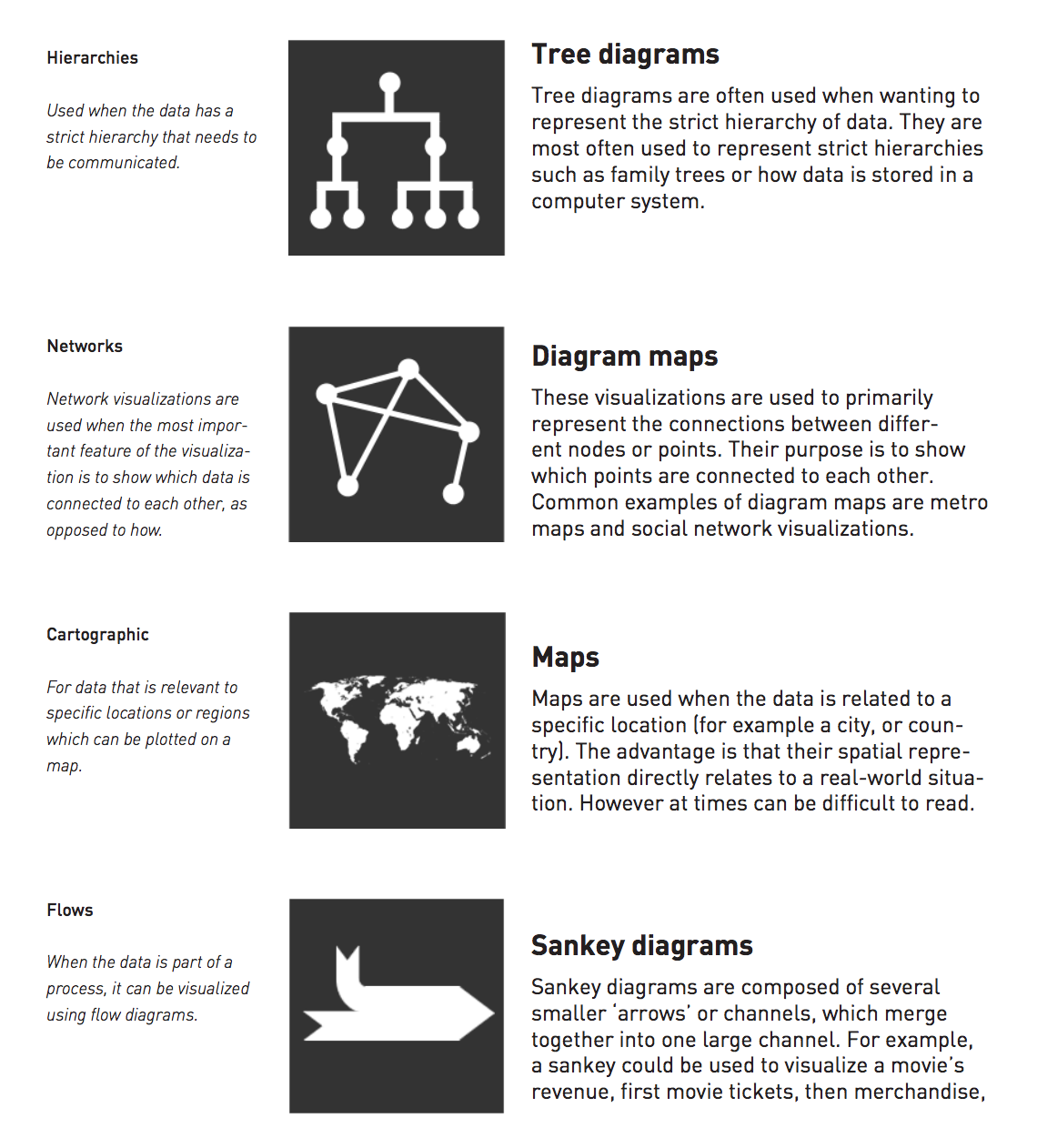(Source: Joel Laumans, An introduction to visualizing data)

Visualizing multi-dimensional data

Univariate data (1D)

1. Line plot2. Bar plot3. Box-and-whisker plot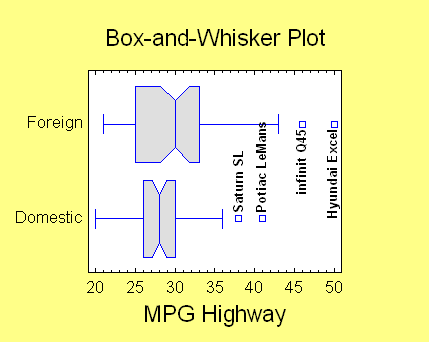Bivariate data (2D)

1. 2D scatter plotTrivariate data (3D)

1. Use 3D scatter plot2. Map two variables [x, y] in 2D space + Map third variable [z] with another visual attribute (ex: color, shape, size)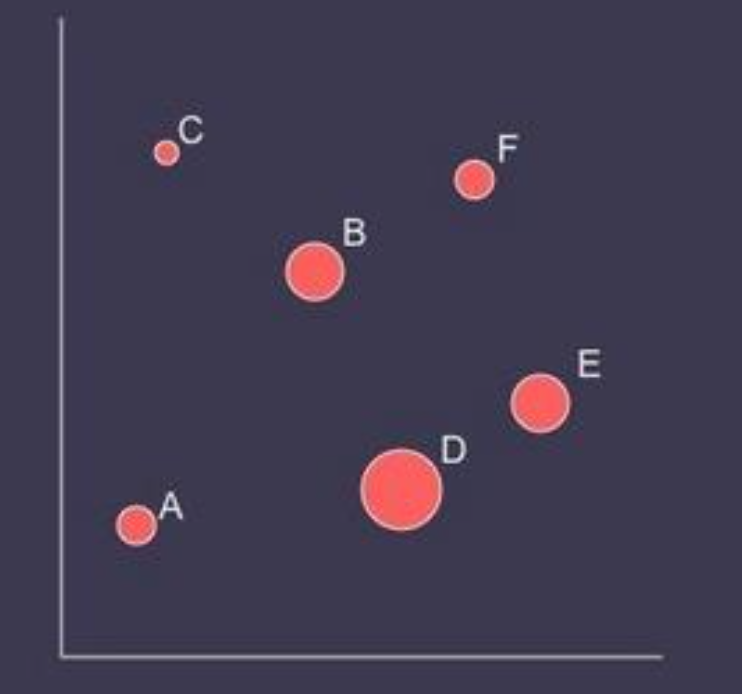Multivariate data (>3D)

• How many variables can be depicted in a image?

"With up to three rows, a data table can be constructed directly as a single image. However, an image has only three dimensions. And this barrier is impassible." -- Bertin

• Example: The wealth and health of nations by year (4 variables)
• See Hans Rosling's "The joy of stats", which visualized the data with animations. (동적 시각화)
• Also see Mike Bostock's interactive version (인터랙티브 시각화)

In-class Practice: Worldwide Disasters (1900-2008)

• Visualize with the data below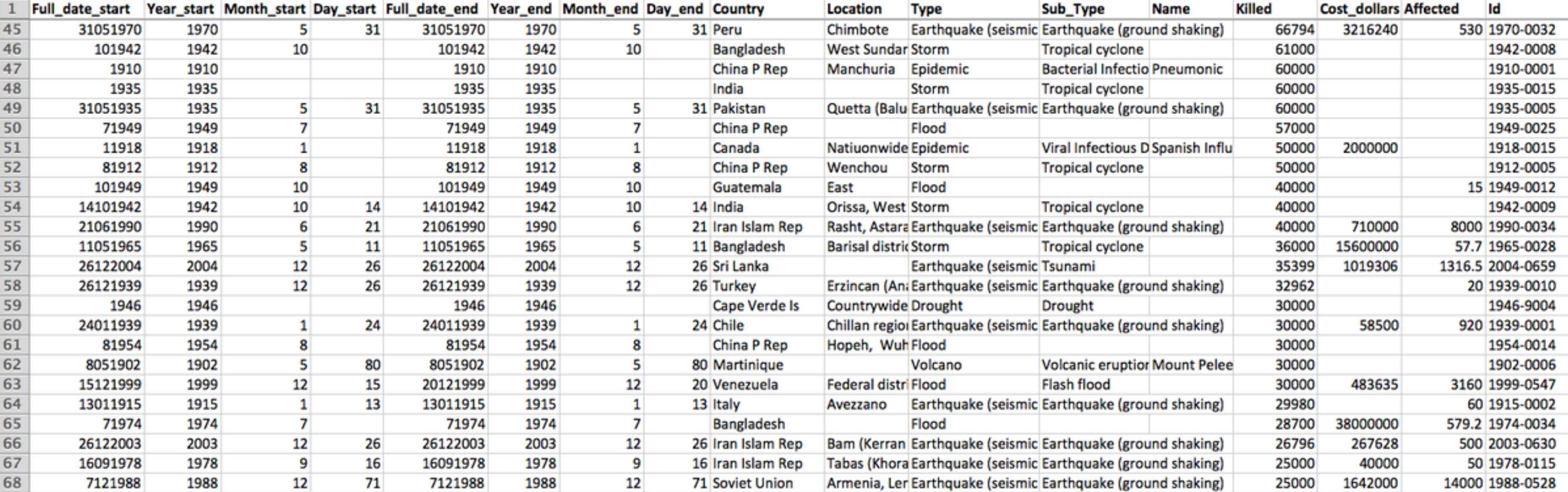• Evaluation
• Expressiveness
• Do the mappings show the facts and only the facts?
• Are visual mappings consistent? (e.g., respect color mappings)
• Effectiveness
• Are perceptually effective encodings used?
• Are the most important data mapped to the most effective visual variables?